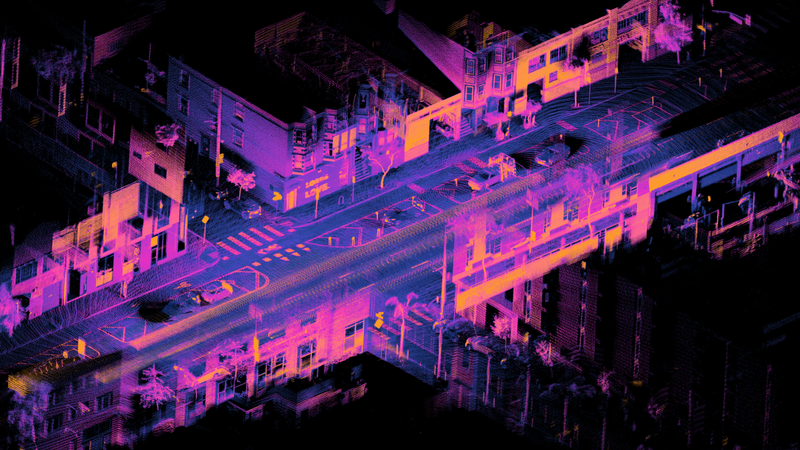# Stereo Vision, Neural Nets and Demand in Autonomous Vehicles

Original article can be found here (source): Artificial Intelligence on Medium# Motivation to Use Geometry for Parallax Error

Using both eyes and staring at an object heads-on creates depth because our own vision appears to converge. It’s similar to a perspective photo where all parallel lines intersect at some far-off horizon point.

Images taken from a camera, however, cannot create depth like our eyes can.

An image transforms a 3D view of the world into a 2D projection. More formally this results in a line transforming into a single point. In Euclidean Space, which is the geometric space contained in a 2D plane, parallel lines cannot intersect like they do in a perspective image (which belongs to Projective Space). This is indicated by the line OX shown to the left, although it corresponds to unique points at varying distances, the camera cannot tell. From the camera’s perspective, it sees a single point x instead of the line OX.

Cameras cannot communicate with one another like our brain can, to assist our eyes in producing images. This results in two flat projections of the same image, taken at different angles. All depth information has been lost. However, by triangulating both projections, applying some geometry, and using parallax error, cameras can be related to one another to solve for distance analytically.

The dual-camera setup shown above is the basis for depth estimation, it is referred to as stereo vision.

# Mathematically Estimating Depth

Deriving and quantifying the parallax effect for depth is math-intensive. If you are only interested in the main formula, skip to the end of this sub-section.

Refer to the diagram above in the previous sub-section for the derivation.

Assume both cameras are located at centers O and O’ which converge at X.

The line OX appears as a single point, x, on the left projection plane. However, when viewed from the right projection plane, there is a set of corresponding points, x’, that form a line in the plane called the epipole, l’. This indicates depth and proves the point x is actually a line OX; now the task is to determine where x is.

Special lines called epilines can be drawn along OX, to converge at O’. The point x can be found by checking each epiline until one that intersects x is found, this solution is the epipolar constraint. Multiple indications of X, each matching a specific epiline, imply possible solutions to the point x.

Now the problem can be redrawn from a top-down view.

Given a particular solution to epipolar constraint, x, its corresponding point in the opposite plane, x’, the line-of-sight OX and the epipolar line for the opposite camera, O’X, depth can be solved.

The points x and x’ are at different depths.

Both lines, OX and O’X are at different angles, yet can be related by equivalent triangles which is why the difference (x — x’) is equal to parameters: B, f, Z (in equation below). That difference of (x — x’) is disparity: a numerical measurement of the parallax error.

More formally disparity refers to the distance in coordinates of two similar regions (as seen from either image projection). Disparity is inversely related to the depth, Z, proven by solving the equivalent triangle relation.

For objects that are closer, parallax error is more pronounced, indicating a large disparity as the same point appears in different locations from both projections. Due to the inverse relationship, it must correspond to a short depth.

Verify the above assumption with the experiment shown in an earlier section. Your observation should match up with the mathematical relation defined.

The value of x is solved via the epipolar constraint, and x’ is known from checking opposite camera’s image projection. Given some distance between cameras, B, and focal lengths, f, then Z can be calculated.

Applying this across all pixels of a scene produces a disparity map, that indicates depth by colour.

For more information on stereo vision and 3D reconstruction, visit OpenCV’s documentation and Dr. Rich Radke’s ECE6969 course.